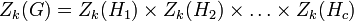# Upper central series may be tight with respect to nilpotency class

Jump to: navigation, search

## Statement

Let$c$ be any natural number. Then, we can construct a Nilpotent group (?)$G$ with the following property.

Let$Z_k(G)$ denote the$k^{th}$ member of the Upper central series (?) of$G$:$Z_1(G) = Z(G)$ is the center and$Z_k(G)/Z_{k-1}(G)$ is the center of$G/Z_{k-1}(G)$ for all$k$. By definition of Nilpotency class (?),$Z_c(G) = G$.

We can find a$G$ with the property that for any$k \le c$, the upper central series of$Z_k(G)$ is precisely the first$k$ terms of the upper central series of$G$. In particular, we can find a$G$ with the property that each$Z_k(G)$ has nilpotence class precisely$k$: in other words, it is not a group of class$k - 1$.

## Related facts

The corresponding statement is not true for the lower central series. Some related facts:

## Proof

Let$H_1, H_2, \dots H_c$ be groups such that each$H_k$ is a nilpotent group of nilpotency class precisely$k$, i.e., it is not nilpotent of class smaller than$k$. Define$G$ as the external direct product:$G = H_1 \times H_2 \times \dots \times H_c$

Now, for each$k$, we have:$Z_k(G) = Z_k(H_1) \times Z_k(H_2) \times \dots \times Z_k(H_c)$

In particular, we obtain that:$Z_k(G) = H_1 \times H_2 \times \dots \times H_k \times Z_k(H_{k+1}) \times \dots \times Z_k(H_c)$

From the given data, in particular the fact that$H_k$ has nilpotency class exactly$k$, it is clear that$Z_k(G)$ has nilpotency class exactly$k$.